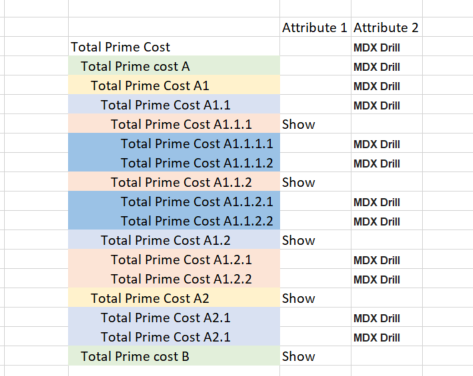# MDX to show different hierarchy level

Hi Expert,

I have a chart where the Account dimension linked to global filter as below:

[Account].[Account].[\$<<tm1.Account.Account].Children

Here’s the Hierarchy:How would I make changes in the above MDX to achieve the below:

1, When I choose “Total Prime Cost” - it will display whatever marked as “Show”
2, When I choose the other accounts - it will show its children.

I found an MDX reference guy in tm1forum where it might be possible to use Case, When, Else, End but not too sure how it works in Apliqo
https://www.tm1forum.com/viewtopic.php?t=16217

Thank you very much!

To achieve this you would first need to store in the TM1 model the elements which should be displayed. You would normally do this by creating an attribute in the Account dimesion. For argument’s sake let’s assume that this attribute is called “Show” and contains the value “Show” when an element should be displayed in your use case.

For the first case the MDX would be

``````{Filter(
{Descendants([Account].[Account].[\$<<tm1.Account.Account>>])},
[Account].[Account].CurrentMember.Properties("Show") = "Show"
)}
``````

For the 2nd case the MDX is what you already have.

``````{[Account].[Account].[\$<<tm1.Account.Account>>].Children}
``````

The problem is combining both these statements as both IIF and CASE/THEN/ELSE in MDX can only return objects or values and NOT members or sets. Maybe some brainiac can solve this with pure MDX. However, you could solve this by having a 2nd attribute in the Account dimension called “MDXDrill” in addition to “Show”.

Against the MDXDrill attribute against each account element you would store a string exactly as the 1st case above against “Total Prime Cost” and the 2nd case against all other elements. (Maybe for leaves you might want to change it to just `{[Account].[Account].[\$<<tm1.Account.Account>>]}` as .children would return empty. Then against the Account dimension on rows (not filter) you would just make the list type “MDX” and the MDX would sumply be `\$<<tm1.Account.Account::MDXDrill>>` As you can nest setting service variables within each other this should work just fine and achieve what you want it to.

@cw-ch

Thank you very much! Sorry I dont quite get what you meantCould I please just elaborate that you mean, There should be 2 attribute like this:I’m not sure how I should write the MDX code. I’m so sorry

Attribute 1 Attribute 2
Total Prime Cost MDX Drill
Total Prime cost A MDX Drill
Total Prime Cost A1 MDX Drill
Total Prime Cost A1.1 MDX Drill
Total Prime Cost A1.1.1 Show
Total Prime Cost A1.1.1.1 MDX Drill
Total Prime Cost A1.1.1.2 MDX Drill
Total Prime Cost A1.1.2 Show
Total Prime Cost A1.1.2.1 MDX Drill
Total Prime Cost A1.1.2.2 MDX Drill
Total Prime Cost A1.2 Show
Total Prime Cost A1.2.1 MDX Drill
Total Prime Cost A1.2.2 MDX Drill
Total Prime Cost A2 Show
Total Prime Cost A2.1 MDX Drill
Total Prime Cost A2.1 MDX Drill
Total Prime cost B Show

I already said one way to make this work. Please do the training! It is all online and available to you. Training Materials - Apliqo UX Documentation - Confluence literally all you need to do this is the demo application which can be installed automatically by the installer. Think of it as an investment.

Provided you explained the requirements correctly then exactly what is below is the setup which you would need.

Show MDXDrill
Total Prime Cost {Filter({Descendants([Account].[Account].[\$<<tm1.Account.Account>>])}, [Account].[Account].CurrentMember.Properties(“Show”) = “Show”)}
Total Prime cost A {[Account].[Account].[\$<<tm1.Account.Account>>].Children}
Total Prime Cost A1 {[Account].[Account].[\$<<tm1.Account.Account>>].Children}
Total Prime Cost A1.1 {[Account].[Account].[\$<<tm1.Account.Account>>].Children}
Total Prime Cost A1.1.1 Show {[Account].[Account].[\$<<tm1.Account.Account>>].Children}
Total Prime Cost A1.1.1.1 {[Account].[Account].[\$<<tm1.Account.Account>>].Children}
Total Prime Cost A1.1.1.2 {[Account].[Account].[\$<<tm1.Account.Account>>].Children}
Total Prime Cost A1.1.2 Show {[Account].[Account].[\$<<tm1.Account.Account>>].Children}
Total Prime Cost A1.1.2.1 {[Account].[Account].[\$<<tm1.Account.Account>>].Children}
Total Prime Cost A1.1.2.2 {[Account].[Account].[\$<<tm1.Account.Account>>].Children}
Total Prime Cost A1.2 Show {[Account].[Account].[\$<<tm1.Account.Account>>].Children}
Total Prime Cost A1.2.1 {[Account].[Account].[\$<<tm1.Account.Account>>].Children}
Total Prime Cost A1.2.2 {[Account].[Account].[\$<<tm1.Account.Account>>].Children}
Total Prime Cost A2 Show {[Account].[Account].[\$<<tm1.Account.Account>>].Children}
Total Prime Cost A2.1 {[Account].[Account].[\$<<tm1.Account.Account>>].Children}
Total Prime Cost A2.2 {[Account].[Account].[\$<<tm1.Account.Account>>].Children}
Total Prime cost B Show {[Account].[Account].[\$<<tm1.Account.Account>>].Children}

You would have the Account dimension 2x in the view settings

1. In the filter with the list of elements to pick from being the ones in the table
2. in the *rows with the elements to display having list type = MDX and the MDX definition is `\$<<tm1.Account.Account::MDXDrill>>` (verbatim!!)
1 Like

You are a legend! Thank you very muchI will start the training.